# 误差及梯度下降-李宏毅机器学习

## 一、Error的来源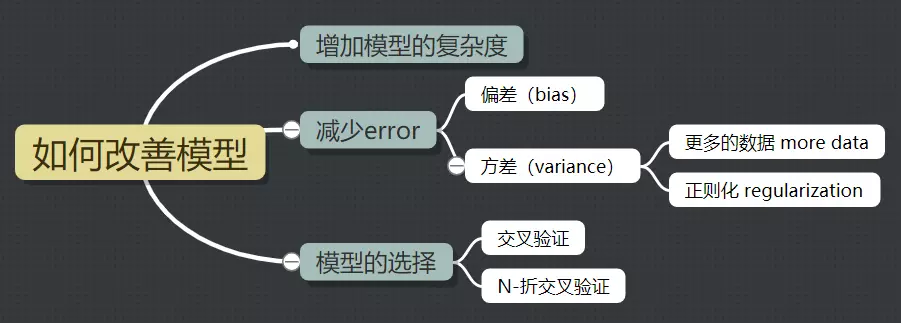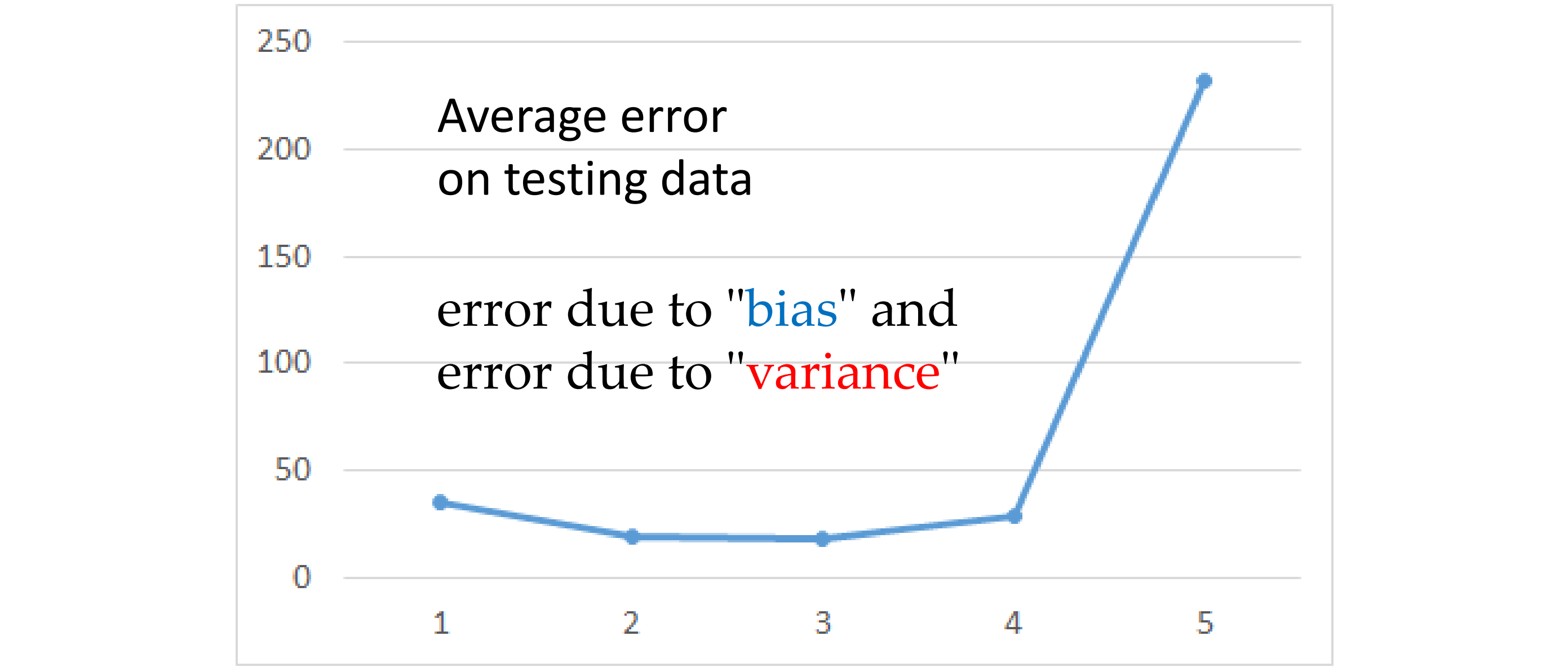### 1.估测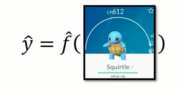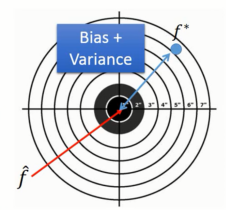#### 估测变量x的偏差和方差

##### 评估x的偏差
• 假设 $x$ 的平均值是 $\mu$，方差为 $\sigma^2$

• 首先拿到 $N$ 个样本点：${x^1,x^2,···,x^N}$
• 计算平均值 $m$, 得到 $m=\frac{1}{N}\sum_n x^n \neq \mu$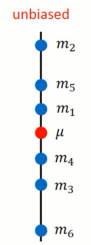$$E[m]=E[\frac{1}{N}\sum x^n]=\frac{1}{N}\sum_nE[x^n]=\mu$$

$$Var[m]=\frac{\sigma^2}{N}$$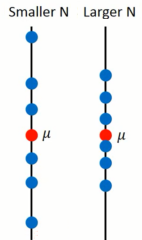##### 估测变量x的方差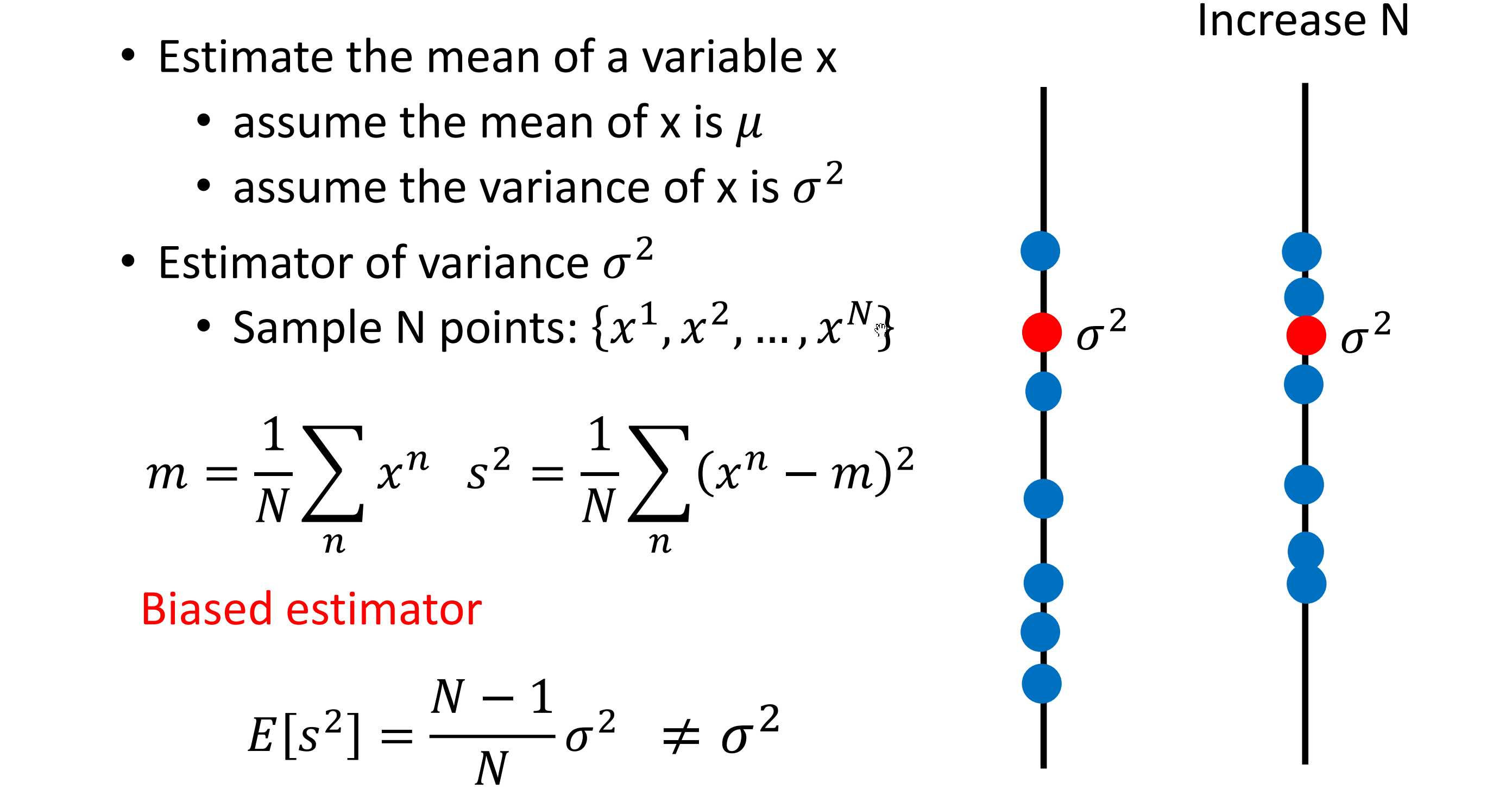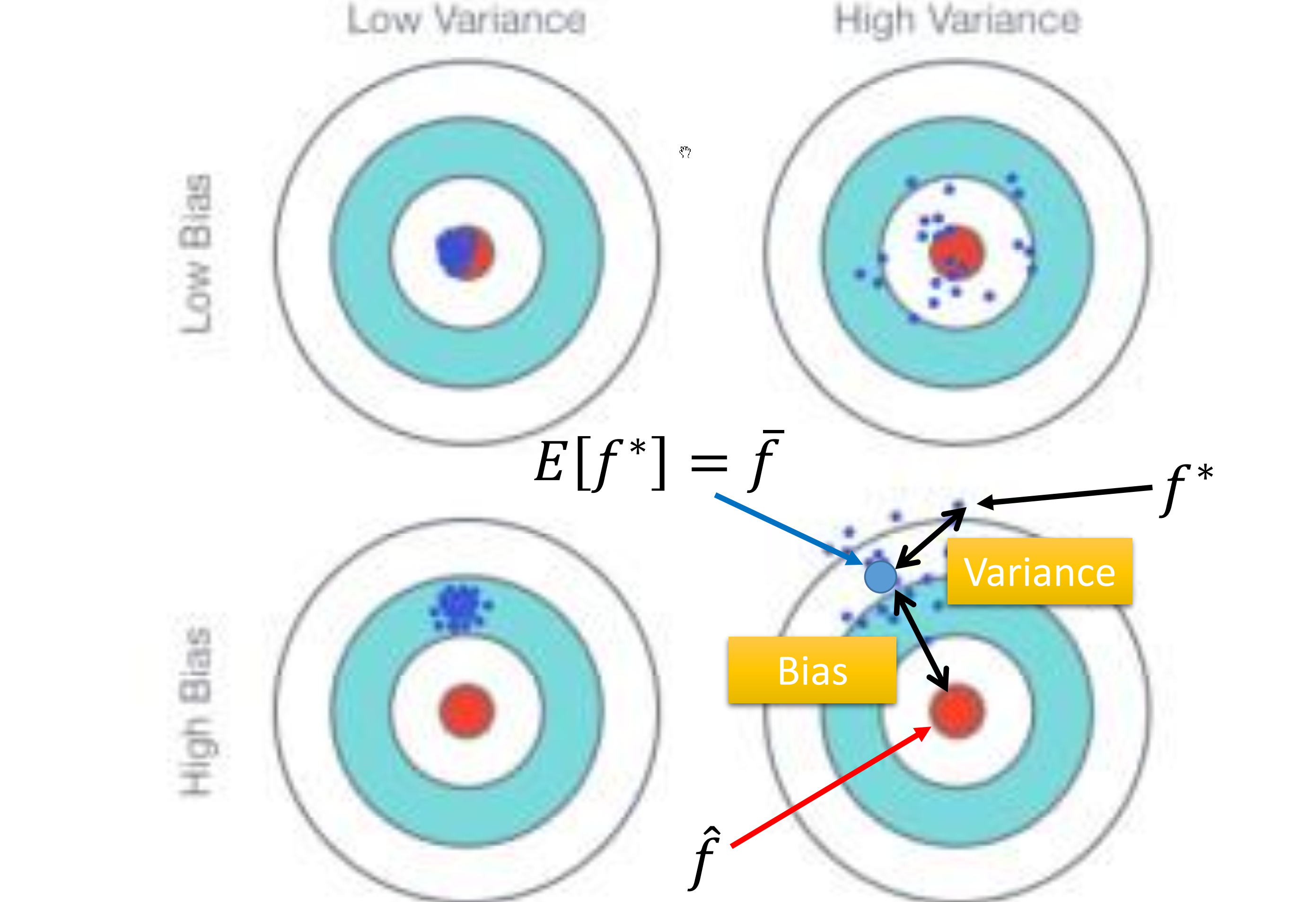#### 为什么会有很多的模型?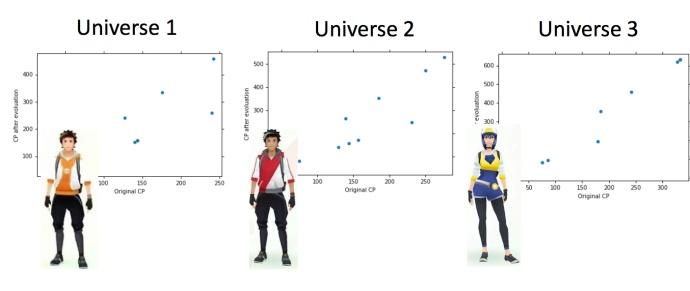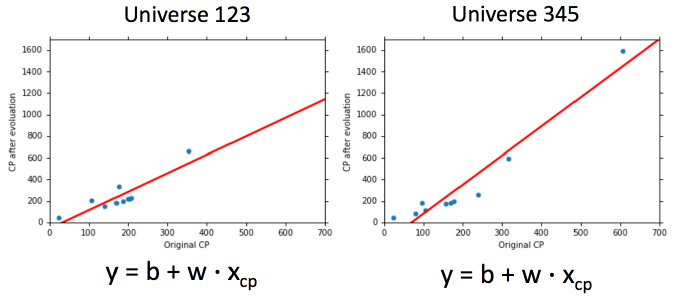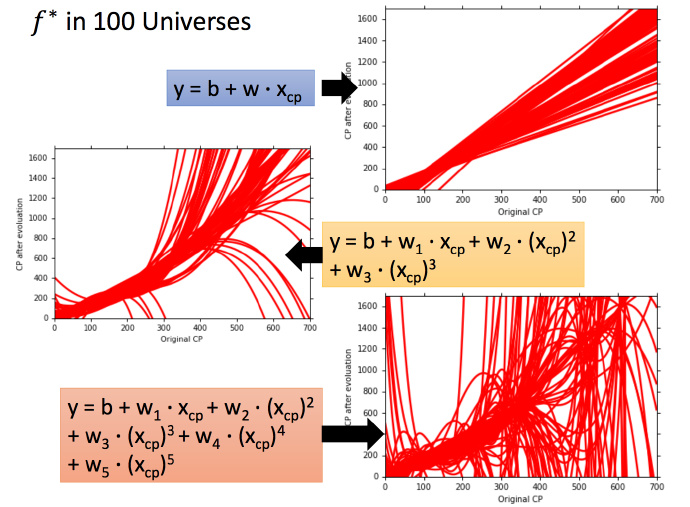##### 考虑不同模型的偏差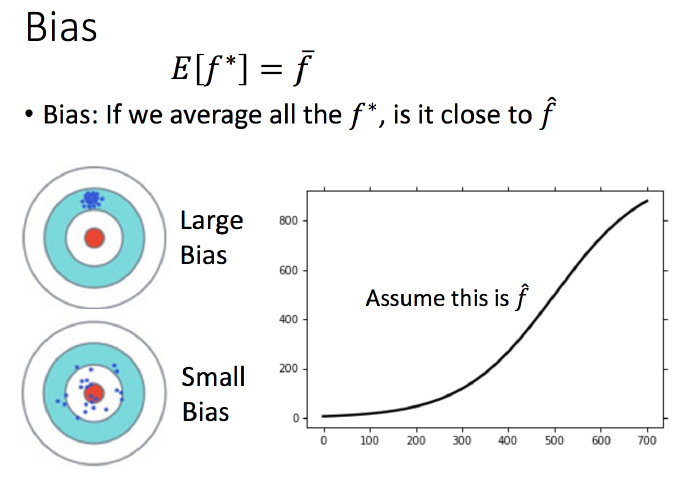##### 偏差v.s.方差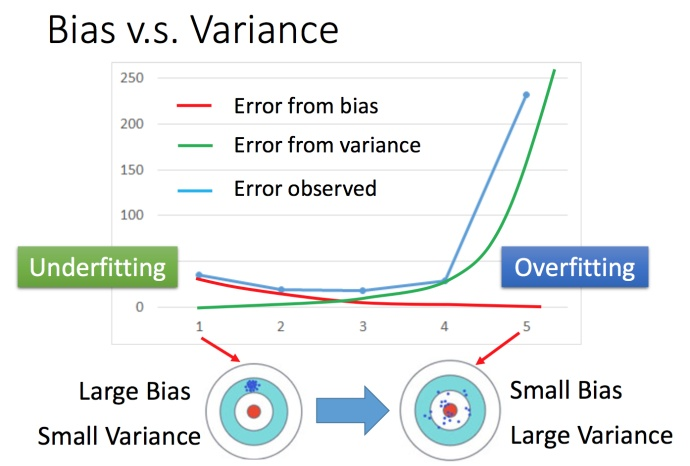### 2.怎么判断？

#### 分析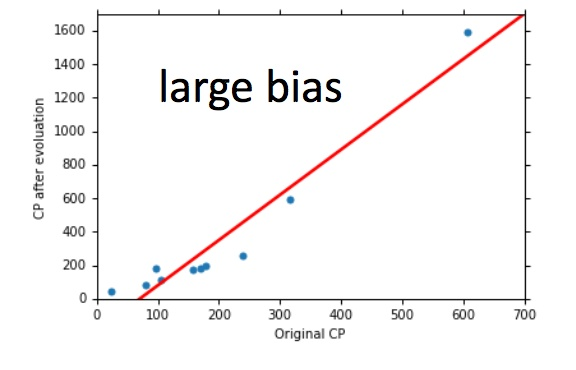##### 方差大-过拟合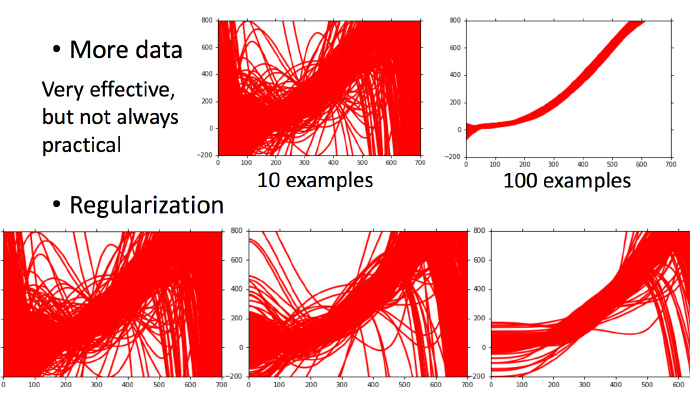### 3.模型选择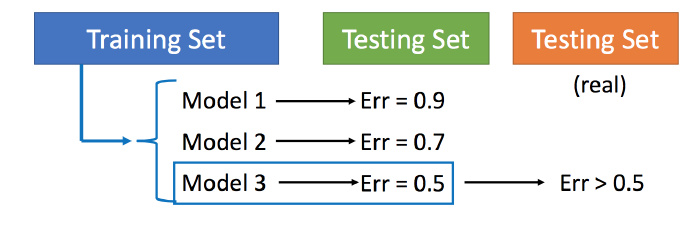#### 交叉验证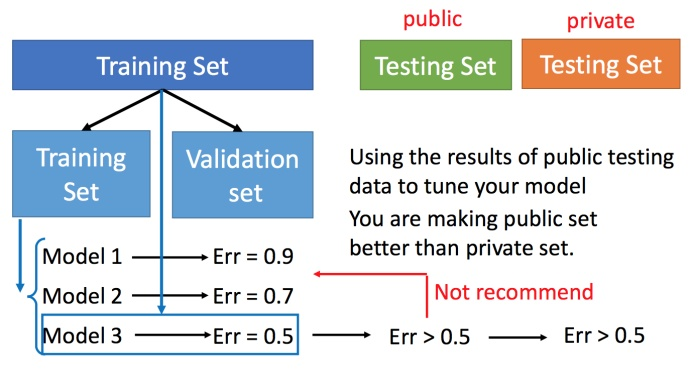#### N-折交叉验证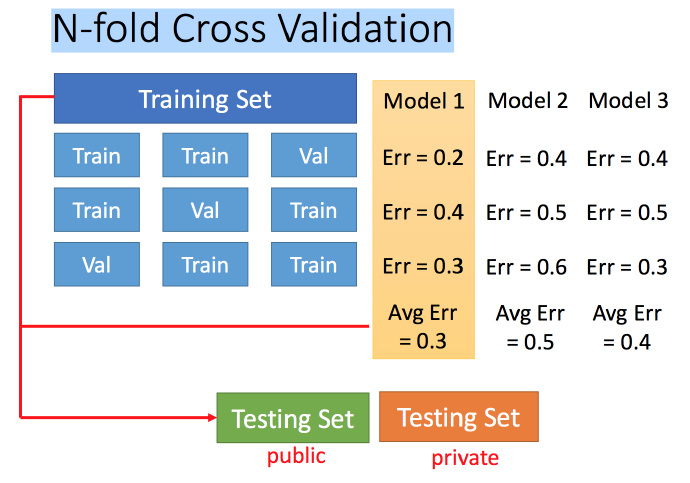## 二、什么是梯度下降法？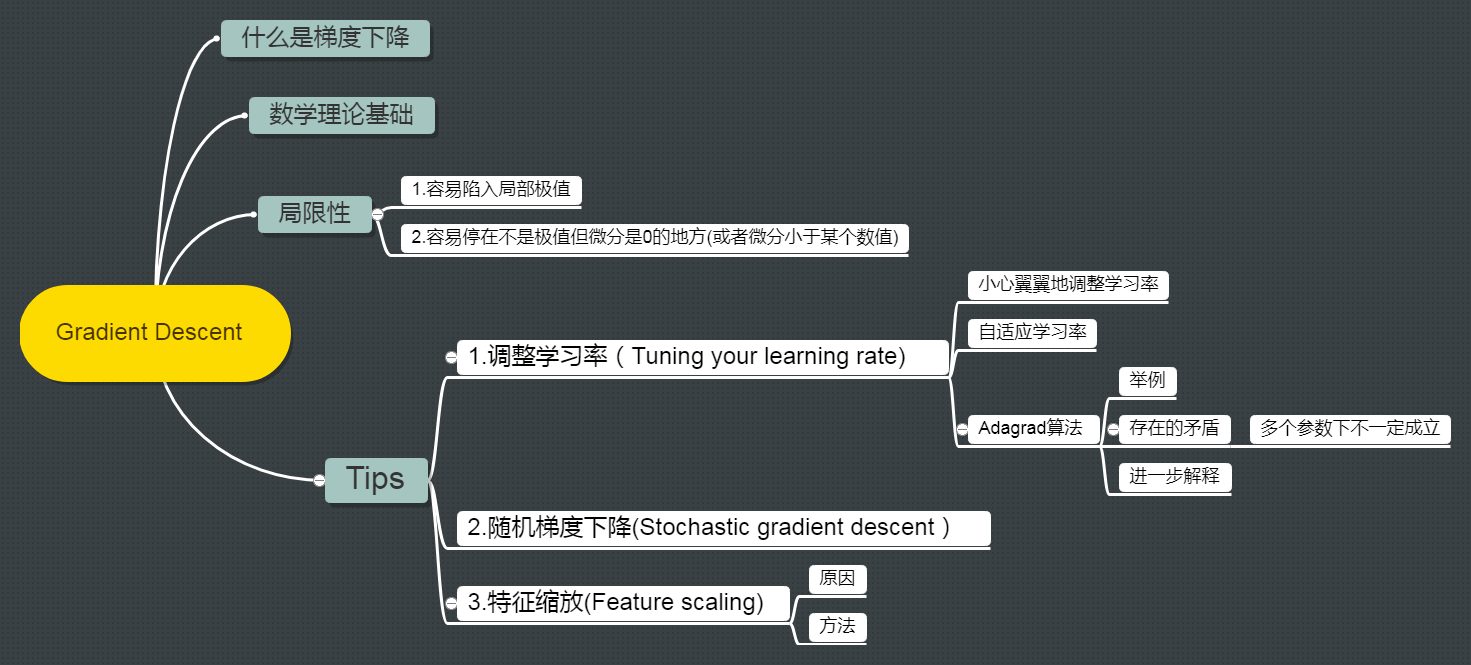#### Review: 梯度下降法

$$\theta^∗= \underset{ \theta }{\operatorname{arg\ min}} L(\theta) \tag1$$

• $L$ :lossfunction（损失函数）
• $\theta$ :parameters（参数）

$$\theta^0 = \begin{bmatrix} \theta_1^0 \ \theta_2^0 \end{bmatrix} \tag2$$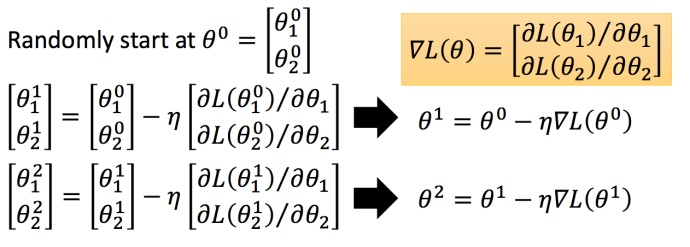$\eta$ 叫做Learning rates（学习速率）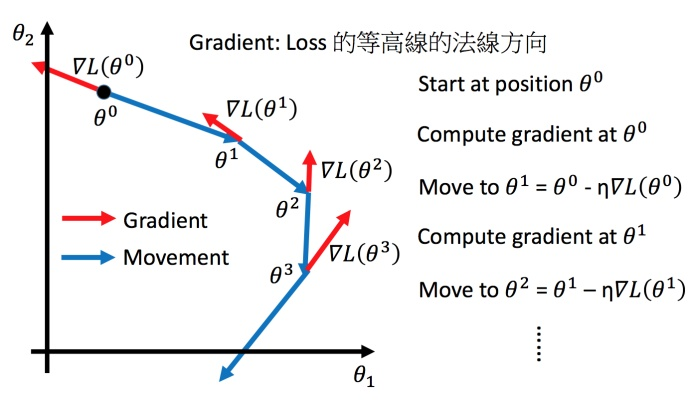### Tip1：调整学习速率

#### 小心翼翼地调整学习率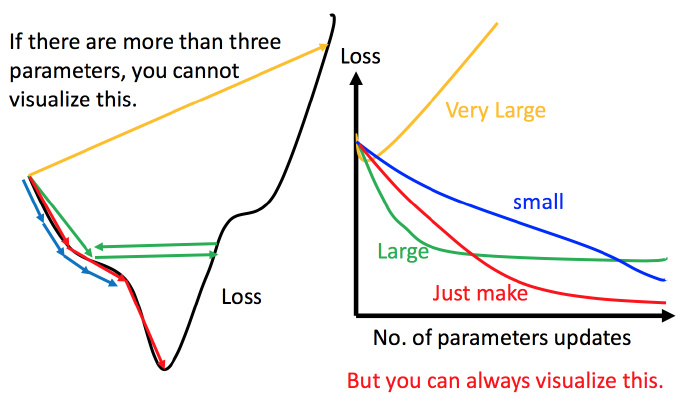#### 自适应学习率

• 通常刚开始，初始点会距离最低点比较远，所以使用大一点的学习率

• update好几次参数之后呢，比较靠近最低点了，此时减少学习率

• 比如 $\eta^t =\frac{\eta^t}{\sqrt{t+1}}$，$t$ 是次数。随着次数的增加，$\eta^t$ 减小

$$w^{t+1} \leftarrow w^t -η^tg^t \tag3$$
$$\eta^t =\frac{\eta^t}{\sqrt{t+1}} \tag4$$

• $w$ 是一个参数

$$w^{t+1} \leftarrow w^t -\frac{η^t}{\sigma^t}g^t \tag5$$
$$g^t =\frac{\partial L(\theta^t)}{\partial w} \tag6$$

• $\sigma^t$ :之前参数的所有微分的均方根，对于每个参数都是不一样的。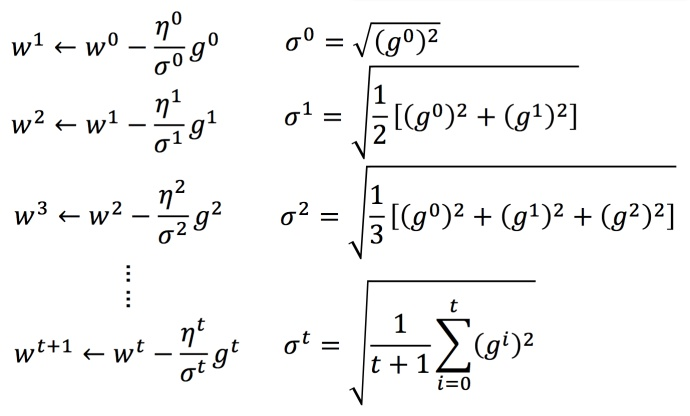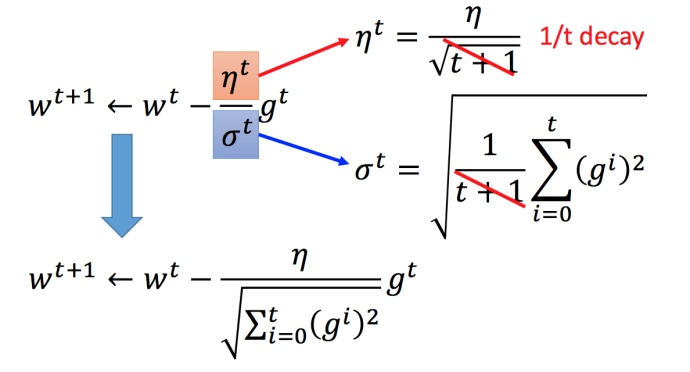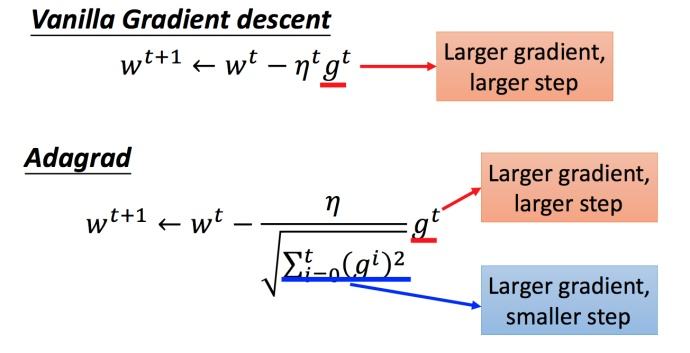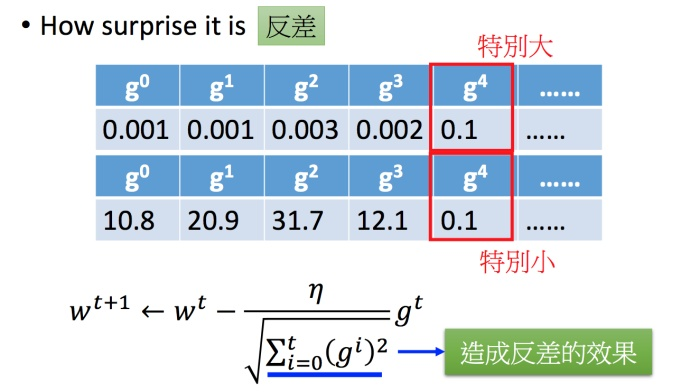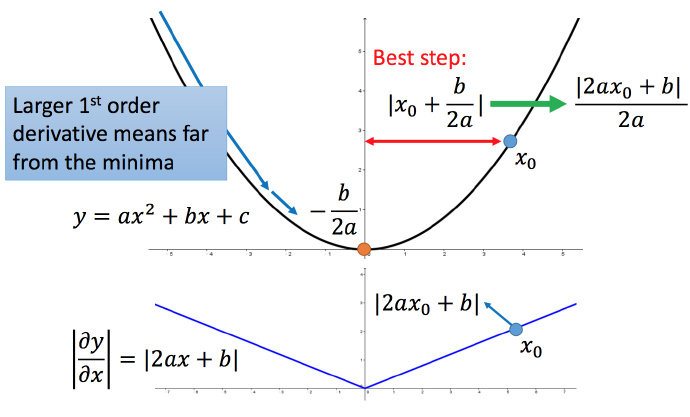##### 多参数下结论不一定成立$$\frac{\partial ^2y}{\partial x^2} = 2a \tag7$$

$$\frac{一次微分}{二次微分}$$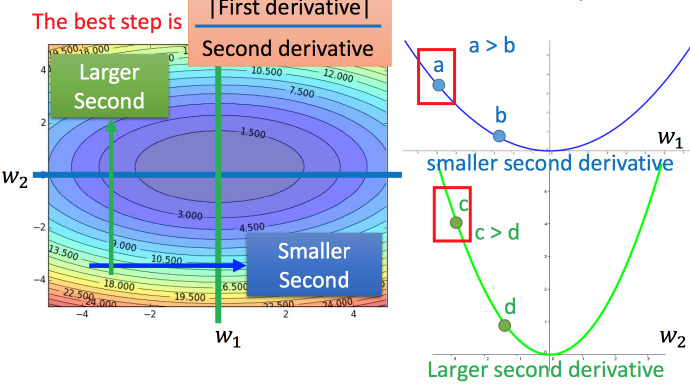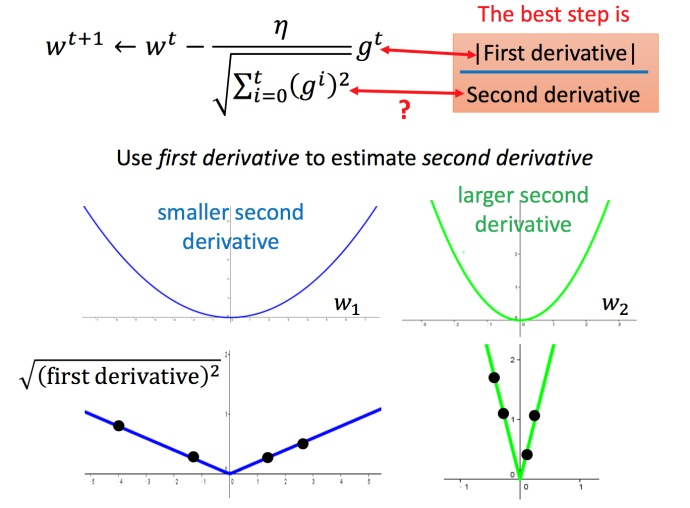### Tip2：随机梯度下降法

$$L=\sum_n(\hat y^n-(b+\sum w_ix_i^n))^2 \tag8$$
$$\theta^i =\theta^{i-1}- \eta\triangledown L(\theta^{i-1}) \tag9$$

$$L=(\hat y^n-(b+\sum w_ix_i^n))^2 \tag{10}$$
$$\theta^i =\theta^{i-1}- \eta\triangledown L^n(\theta^{i-1}) \tag{11}$$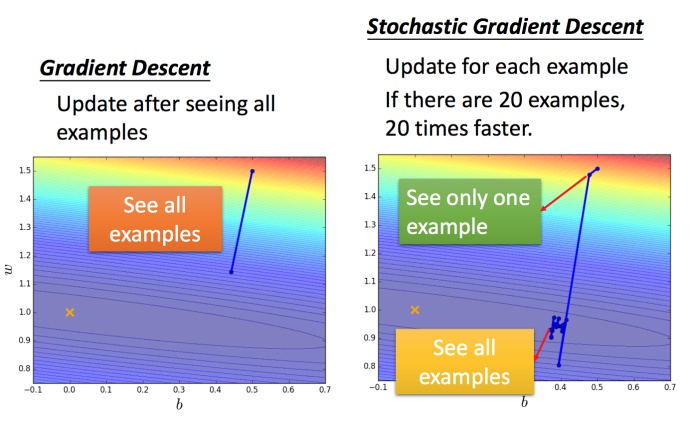### Tip3：特征缩放

$$y=b+w_1x_1+w_2x_2 \tag{12}$$#### 为什么要这样做？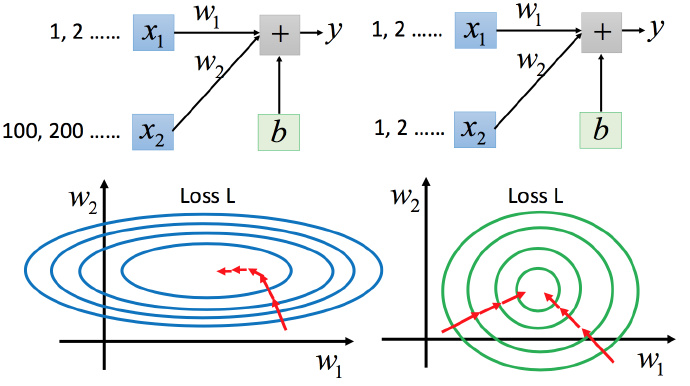#### 怎么做缩放？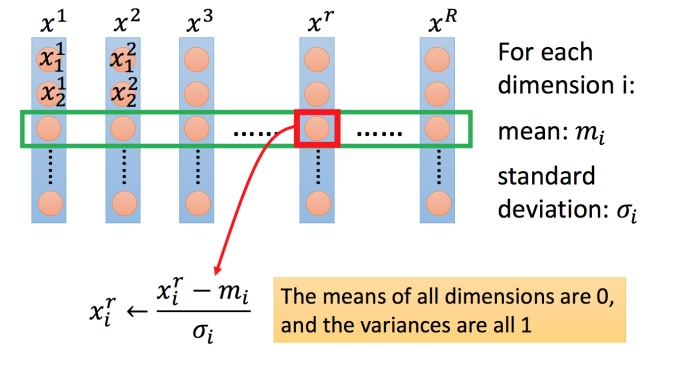### ①梯度下降的理论基础

$$\theta^∗= \underset{ \theta }{\operatorname{arg\ min}} L(\theta) \tag1$$

$$L(\theta^0) >L(\theta^1)>L(\theta^2)>···\tag{13}$$

### ②数学理论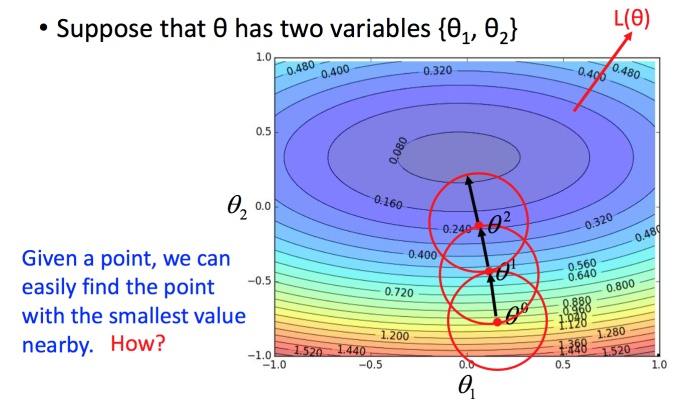#### 泰勒展开式

\begin{aligned} h(x) &= \sum_{k=0}^{\infty }\frac{h^k(x_0)}{k!}(x-x_0)^k \ & =h(x_0)+{h}’(x_0)(x−x_0)+\frac{h’’(x_0)}{2!}(x−x_0)^2+⋯ \tag{14} \end{aligned}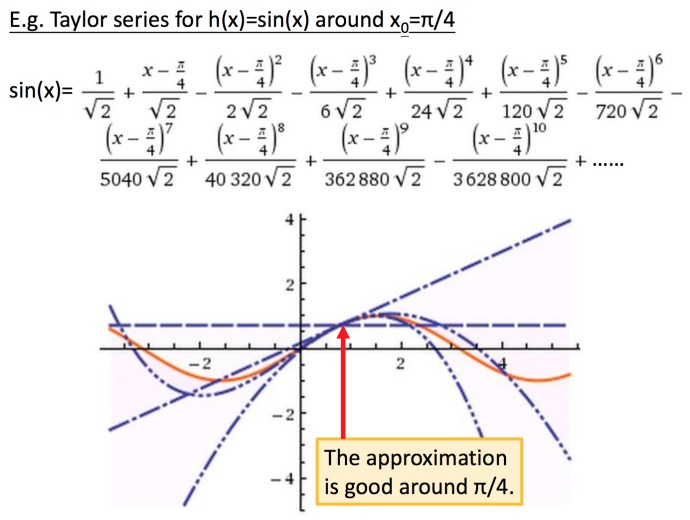##### 多变量泰勒展开式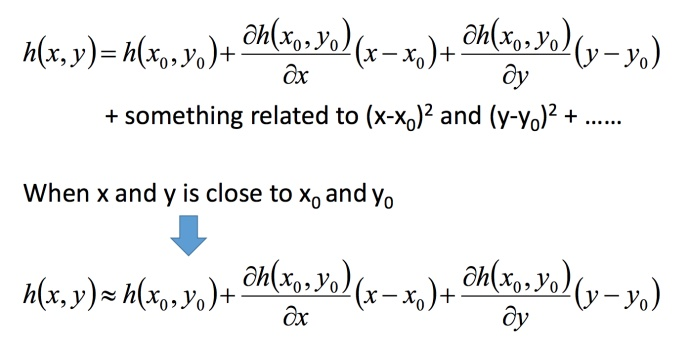#### 利用泰勒展开式简化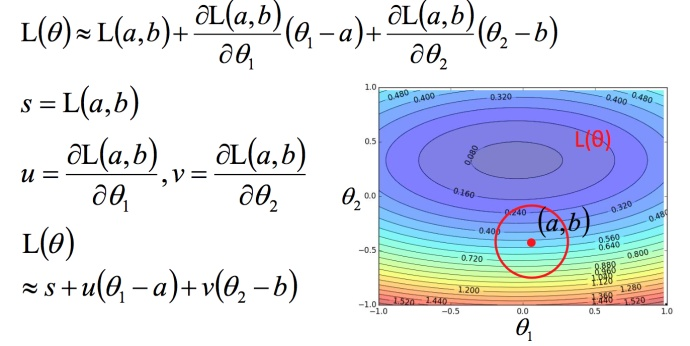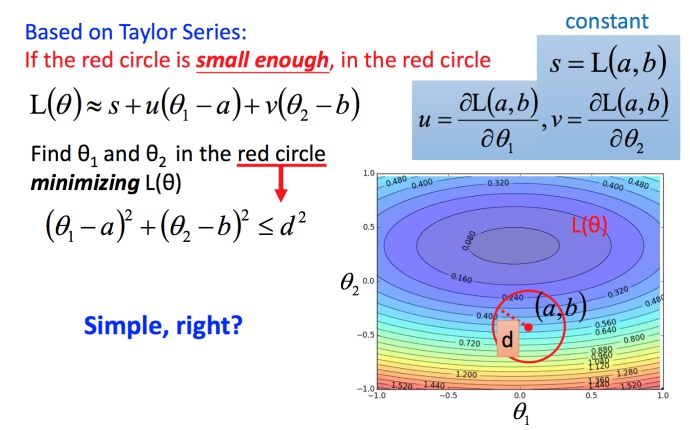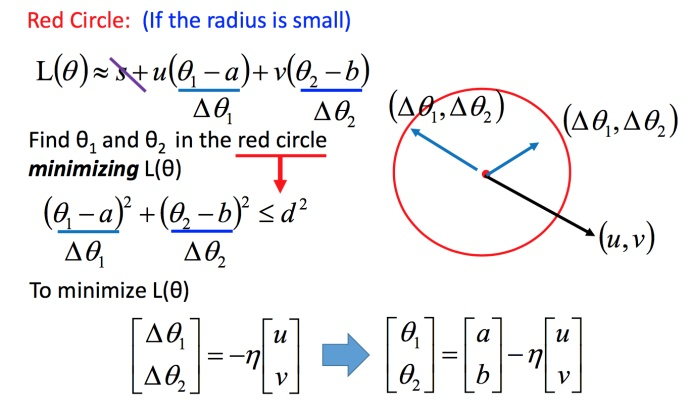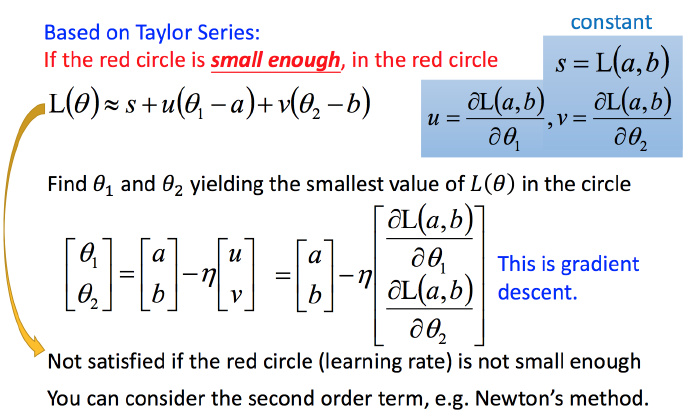$$L(\theta)\approx s+u(\theta_1 - a)+v(\theta_2 - b) \tag{14}$$

### ③梯度下降的限制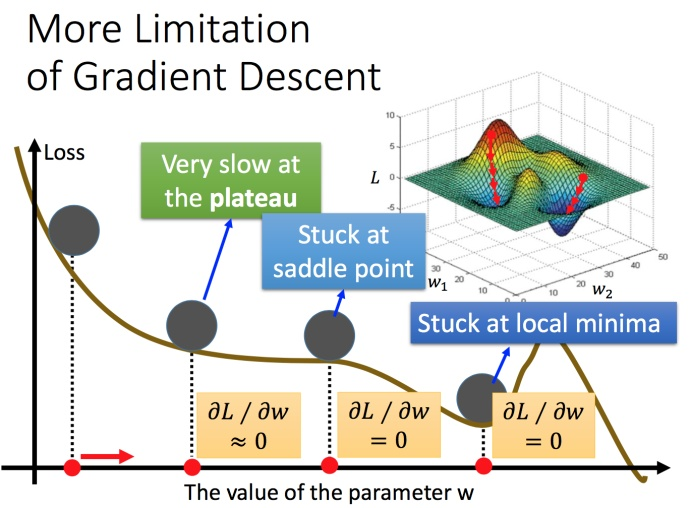## 三、Gradient Descent (Demo by AOE)

• 利用帝国时代的方式模拟梯度下降
• 在地图上大多数位置我们是未知的，只有我们单位走过的地方是可知；
• 地图上的海拔可以看作损失函数loss function，我们的目的就是寻找海拔的最低点的值；
• 随机初始一个位置，朝向较低的方向移动，周而复始，直到local minimal(在不开天眼的情况下，你始终不会知晓所在位置是否为global minimal)。

## 四、Gradient Descent (Demo by Minecraft)

• 利用梯度下降法更新参数，损失函数loss function可能会不降反升(利用Mincraft解释该情况)；
• 人物的前方是较低方向，右方也是较低方向，利用梯度下降法，往右前方移动一步，然后反复用梯度下降法，往右前方移动一步，周而复始；
• 尽管前方和右方是下降的方向，但往右前方移动，将会失败(因为实际右前方是比较高的地方)。

## 参考

https://blog.baixf.tk/2022/07/14/Machine Learning/李宏毅机器学习（含深度学习）/第三章 误差及梯度下降/

2022年7月14日